# QUESTION 3 3 points (E A 2.000-mg sample of a sample is found to have produced...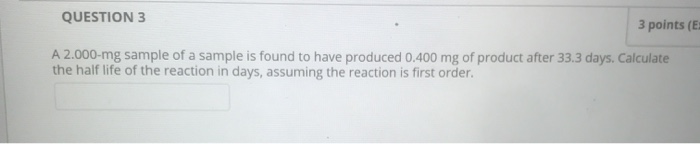QUESTION 3 3 points (E A 2.000-mg sample of a sample is found to have produced 0.400 mg of product after 33.3 days. Calculate the half life of the reaction in days, assuming the reaction is first order.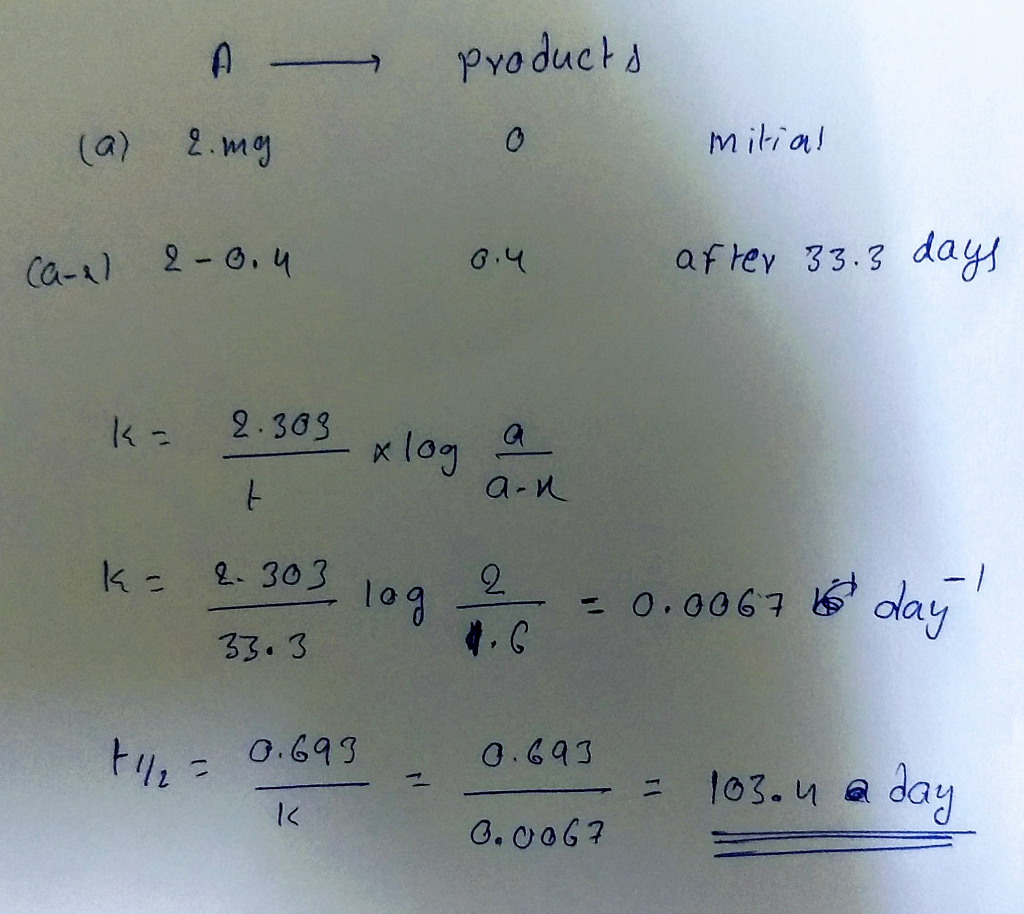##### Add Answer to: QUESTION 3 3 points (E A 2.000-mg sample of a sample is found to have produced...
Similar Homework Help Questions
• ### A The half-life of cobalt-60 is 5.20 yr. How many milligrams of a 2.000-mg sample remains...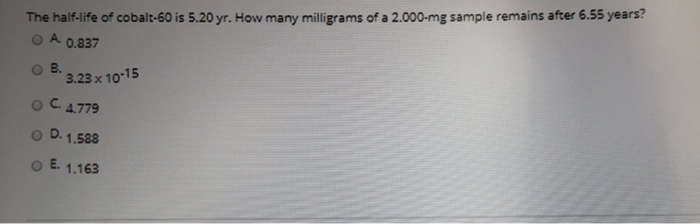A The half-life of cobalt-60 is 5.20 yr. How many milligrams of a 2.000-mg sample remains after 6.55 years? 0.837 B. 3.23 x 10-15 OC. 4.779 OD. 1.588 E. 1.163

• ### 1. For a first order decay of [A], if 525 mg remains of an initial sample...

1. For a first order decay of [A], if 525 mg remains of an initial sample of 1.3079 g after 361 min, what is the half life (in minutes)? 2. The decay of antimony-131 is first order with a half life is 23.03 minutes. How much of 1.4228 g sample would remain after 1.368 hours? 3. The decay of antimony-131 is first order with a half life is 23.03 minutes. How long (in minutes) would it take for a 0.8893...

• ### please show work An aqueous suspension of drug X was formulated containing 100 mg of the...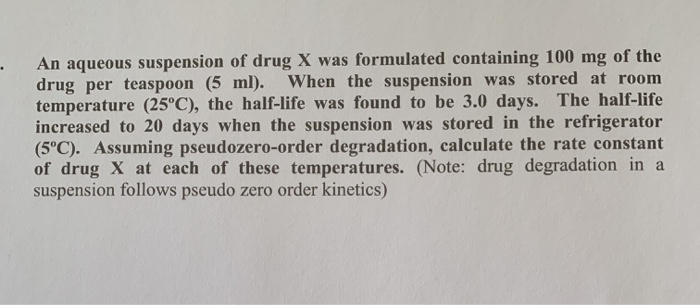please show work An aqueous suspension of drug X was formulated containing 100 mg of the drug per teaspoon (5 ml). When the suspension was stored at room temperature (25°C), the half-life was found to be 3.0 days. The half-life increased to 20 days when the suspension was stored in the refrigerator (5°C). Assuming pseudozero-order degradation, calculate the rate constant of drug X at each of these temperatures. (Note: drug degradation in a suspension follows pseudo zero order kinetics)

• ### Question 14 1 pts A 4.50-mg sample of a newly discovered radioactive nuclide was analyzed and...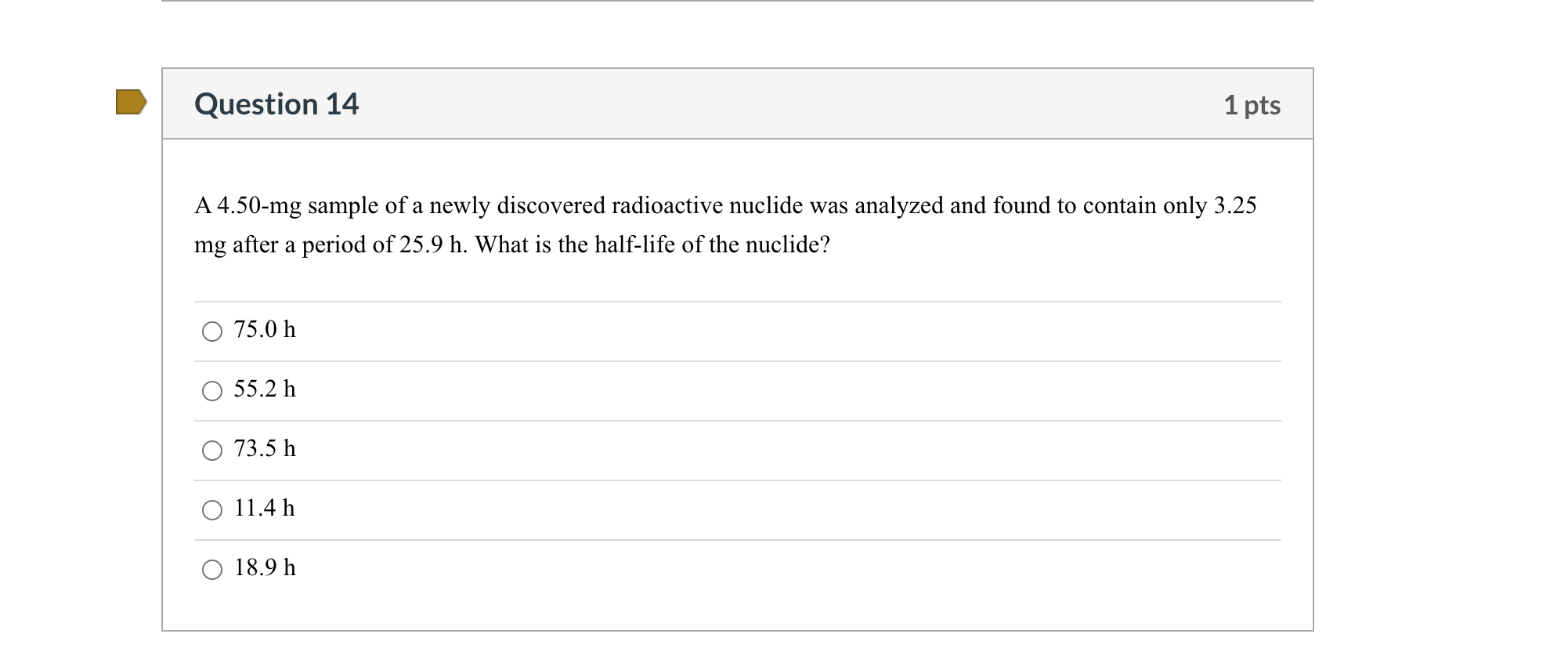Question 14 1 pts A 4.50-mg sample of a newly discovered radioactive nuclide was analyzed and found to contain only 3.25 mg after a period of 25.9 h. What is the half-life of the nuclide? O 75.0 h O 55.2 h O 73.5 h O 11.4 h 18.9 h

• ### Question 1 10 points Save Answer A 0.455 g sample of magnesium is allowed to burn...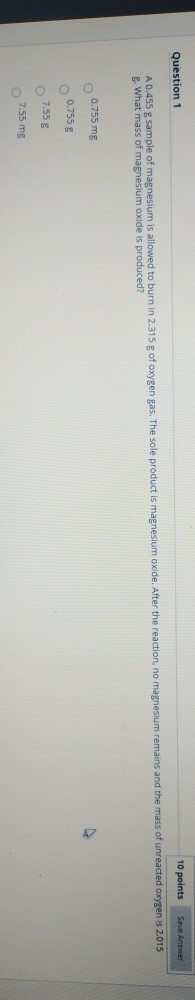Question 1 10 points Save Answer A 0.455 g sample of magnesium is allowed to burn in 2.315 g of oxygen gas. The sole product is magnesium oxide. After the reaction, no magnesium remains and the mass of unreacted oxygen is 2.015 g. What mass of magnesium oxide is produced? 0.755 mg 0.755 g 7.55g 7.55 mg

• ### The half-life for the process 238U→206Pb is 4.5×109yr. A mineral sample contains 61.0 mg of 238U...

The half-life for the process 238U→206Pb is 4.5×109yr. A mineral sample contains 61.0 mg of 238U and 14.5 mg of 206Pb. What is the age of the mineral? To calculate the age of the mineral, begin by rearranging the first-order relationship between the half-life, t1/2, and the decay constant to solve for the decay constant, k. k=0.693/t1/2 Then, use the following formula to solve for the age of the mineral, t: t=−1klnNtN0 where N0 is the original amount of 238U...

• ### Question 8:   (a) (4 points) The reaction A(aq) → B(aq) is a first order reaction with...

Question 8:   (a) (4 points) The reaction A(aq) → B(aq) is a first order reaction with respect to A(aq). The concentration of A(aq) after 100.0 seconds of reaction is 0.960 M and the concentration of A(aq) after 400.0 seconds of reaction is 0.734 M. What will be the concentration of A(aq) after 832.8 seconds of reaction?   (b) (4 points) The reaction A(aq) → B(aq) + C(aq) is a first order reaction with respect to A(aq). The half-life of A(aq) is...

• ### Question 1 (5 points) The half-life of Fluorine-20 is 11.Os. 1) If you have a fluorine-20...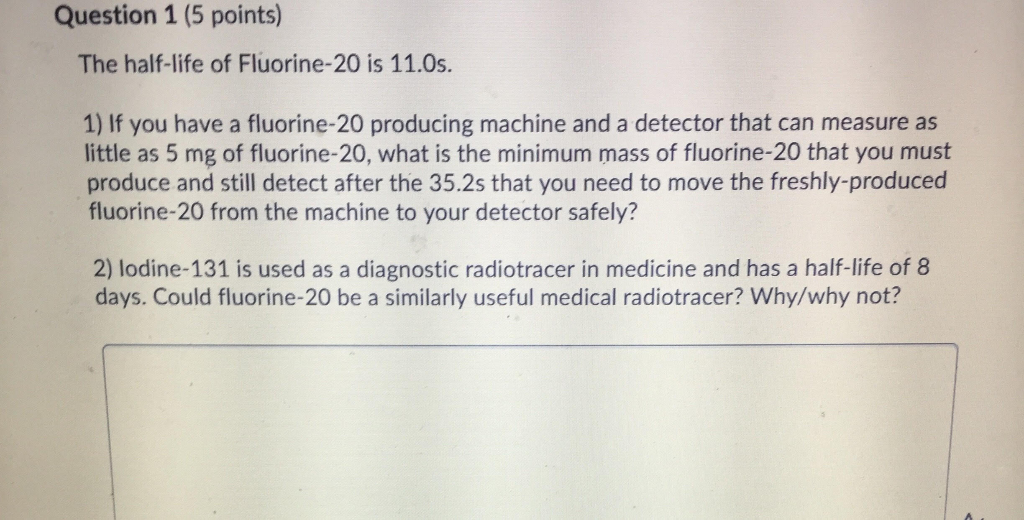Question 1 (5 points) The half-life of Fluorine-20 is 11.Os. 1) If you have a fluorine-20 producing machine and a detector that can measure as little as 5 mg of fluorine-20, what is the minimum mass of fluorine-20 that you must produce and still detect after the 35.2s that you need to move the freshly-produced fluorine-20 from the machine to your detector safely? 2) lodine-131 is used as a diagnostic radiotracer in medicine and has a half-life of 8 days....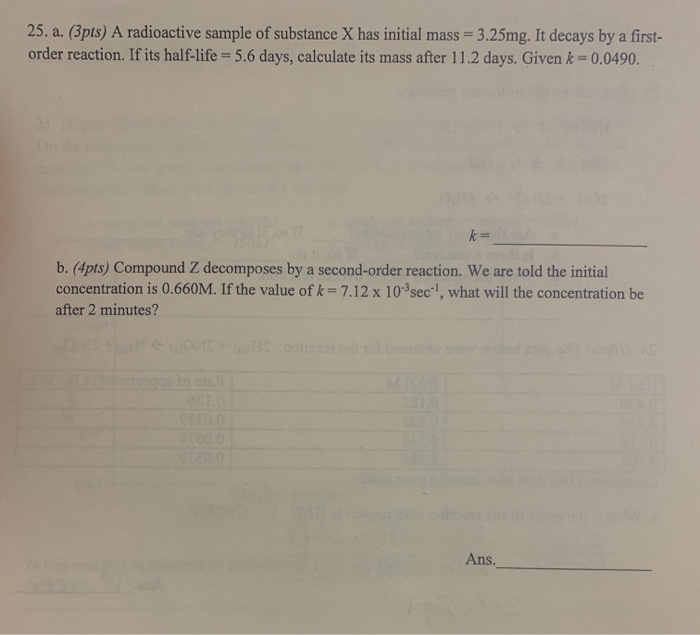please help with question 25 a and b. showing all work 25. a. (3pts) A radioactive sample of substance X has initial mass = 3.25mg. It decays by a first- order reaction. If its half-life = 5.6 days, calculate its mass after 11.2 days. Given k = 0.0490. k= b. (4pts) Compound Z decomposes by a second-order reaction. We are told the initial concentration is 0.660M. If the value of k = 7.12 x 10 sec"!, what will the concentration...

• ### Question 8: (a) (4 points) The reaction A(aq) → B(aq) is a first order reaction with...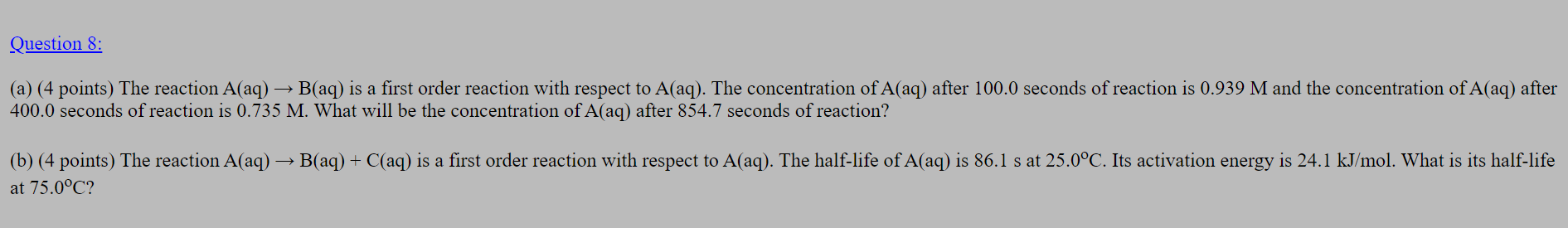Question 8: (a) (4 points) The reaction A(aq) → B(aq) is a first order reaction with respect to A(aq). The concentration of A(aq) after 100.0 seconds of reaction is 0.939 M and the concentration of A(aq) after 400.0 seconds of reaction is 0.735 M. What will be the concentration of A(aq) after 854.7 seconds of reaction? (b) (4 points) The reaction A(aq) → B(aq) + C(aq) is a first order reaction with respect to A(aq). The half-life of A(aq) is...

Free Homework App